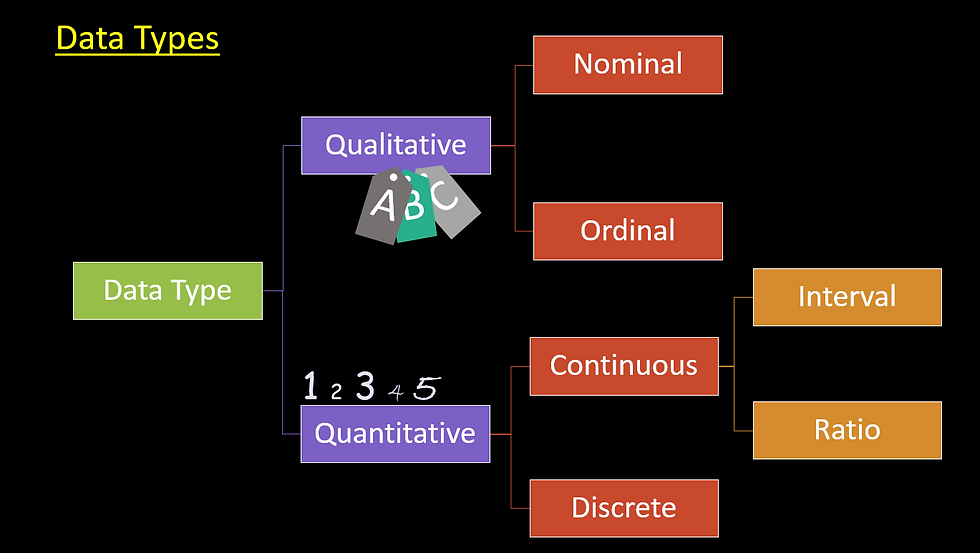top of page
Search

# Statistics Simplified# 1: Data Types

Statistics Simplified is the series to express statistics in layman terms.

Identifying data types is crucial in data analytics. Wisdom says that we should know the data type before we start the data analysis process. And the reasons are apparent. If we understand the data type, then we can apply appropriate mathematical aggregations and statistical tests.

We categorize data into two primary categories: Qualitative & QuantitativeWe can understand data types by the following example:Discrete data types primarily contain count and percentages.

The fundamental difference between a continuous and a discrete data type is that continuous data type is always associated with a unit or a scale, e.g., kilogram, meter, centimeter, degree Celsius, years.

Each data type has its level of measurement:And depending on the data type, we can decide on the underlying mathematical aggregations:Also see: Central Tendency & Variation

#vivran

See All
bottom of page